## Saturday, October 1, 2016

### Latacora Riddle and Browser Fingerprinting

Today on Twitter I stumbled over a tweet by Patrick Thomas (@coffeetocode) where he wrote:
"Hah! I like this. Comment in Latacora source about a cheat is true, but the cheat is way more clever."

with this screenshot: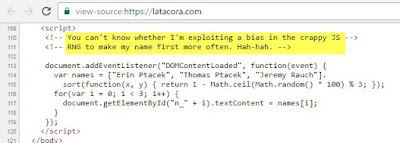Screenshot from @coffeetocode's tweet

﻿﻿This sparked my interest. Looking at the page from the screenshot (latacora.com), I saw that there are three names displayed and this code seems to put the three names (Erin, Thomas and Jeremy) in a random order. But the comment also says that there seems to be some bias. I then saw that the tweet by @coffeetocode also had a second screenshot:
﻿statistics by @coffeetocode

﻿﻿﻿﻿﻿

I did expect some bias due to the fact that there is a calculation of 100%3 which does not equally divide the numbers into three values, but a bias of 60% versus 10% is by far over what I expected, so I had to dig deeper. Here is my analysis.

In the meantime Patrick has posted his own solution, but here my analysis anyway.

The code seems to be very simple. It first declares the names array, then it applies some sort function on it and finally it puts the three names into the HTML page.

I had to look up the documentation about the sort function and I found the w3schools description of it. The function that can optionally be specified is used to sort the array and decides if the two values being passed are greater, smaller or equal. Instead of comparing the values, the function returns a random number.

Let's look at the random number generation first. Everything starts with Math.random(), which is not seeded in any way and if we assume a good browser implementation, this should return some good random values. Maybe not cryptographically secure, but random enough for this purpose.

Math.random() actually returns a number between 0 and 1 (including 0, but not including 1). Multiplying this by 100 should return numbers between 0 and 99.999 (just less than 100). Math.ceil() then rounds this number up to the next integer.

This is actually a fail here, because this will result in numbers between 0 and 100, with 101 different possible values. The value 0.001 will be rounded up to 1 for example, but 0 will remain 0. The value 0 (exactly 0) is probably pretty rare though. The chance that exactly 0 comes back from this code is about the same as if Math.random() would return exactly 0.239183777281903 - it depends on the implemented resolution of the float numbers. I tried this out in the browser and exactly 0 was never returned, so I'll ignore this for the following investigation, but a correct implementation would not use Math.ceil(), but Math.floor()+1 instead. If we ignore the 0 which practically does never exist, we now have integer values between 1 and 100.

I then followed a wrong trail by not reading the code correctly. I thought that the next calculation is (1 - calc) % 3, with calc being our random number between 1 and 100. This yielded to negative numbers and there is a known bug in the JavaScript implementation about negative numbers when calculating the modulo. Feel free to look that up too, but this is wrong, there are no brackets, which means the modulo will be calculated first. This means that we are calculating 1 - (calc % 3), which is much simpler. calc % 3 returns values between 0 and 2, and calculating 1 minus this give us values -1, 0 or 1; exactly what the sort function expects.

There is actually already a little bias there. The modulo 1 - calc % 3 returns the numbers -1, 0 or 1 with the following probabilities:
• -1: 33/100
• 0: 34/100
• +1: 33/100
As you can see, the 0 is slightly more frequent than the other two values. This comes from the fact that we have calc in the range of 1 to 100 and both 1 - 1 % 3 equals to 0 and also 1 - 100 % 3 is also 0. For an equal distribution the code should not multiply by 100, but by 99 (or simply by 3) instead. This is just a slight bias that does not contribute much to the end result.

Now the question is what does the sort function do when the compared values are purely random? In order to have consistent results, we would need to know in which order the sort function is called. Let's look at the real documentation:
"If comparefn is not a consistent comparison function for the elements of this array, the sort order is implementation-defined."

There you have it. This means that the sorting is highly implementation dependent. I tried with different browsers and indeed I got different results. But in all cases Erin was always first (with the highest percentage). Let's have a closer look at how the sorting works.

I tried with seven different scripting engines:
• Windows scripting host (cscript)
• Internet Explorer 11 and Edge (both sort identical)
• Chrome, Firefox, Brave (all three sort identical)
• Mobile Safari
It's interesting that cscript always does three calls to the sort function, independent of what was the result of the first two calls. In some cases we even get the same call twice (for the same two names). That seems inefficient. IE and Edge need between two and five calls to the sort function, while all others call the sort function either twice or three times. I do not understand what the advantage would be to sorting this array with five calls.

Anyway, I simulated the sorting in the different engines and created some Excel lists of which results of the sort function yield which sort order. For Chrome, Firefox and Brave we have the following list:
• -1, -1: ETJ (1. Erin, 2. Thomas, 3. Jeremy)
• -1, 0: ETJ
• -1, 1, -1: EJT
• -1, 1, 0: EJT
• -1, 1, 1: JET
• 0, -1: ETJ
• 0, 0: ETJ
• 0, 1, -1: EJT
• 0, 1, 0: EJT
• 0, 1, 1: JET
• 1, -1: TEJ
• 1, 0: TEJ
• 1, 1, -1: TJE
• 1, 1, 0: TJE
• 1, 1, 1: JTE
From this overview you can already see that Erin is quite often first, sometimes Thomas, but rarely Jeremy. Calculating the percentages (taking into account the different probabilities for the three different values) we get:
• Thomas first: 29.406%
• Thomas second: 48.484%
• Thomas third: 22.110%
• Jeremy first: 10.890%
• Jeremy second: 22.110%
• Jeremy third: 67.000%
• Erin first: 59.704%
• Erin second: 29.406%
• Erin third: 10.890%
This matches with the screenshot from the beginning. I also made a script that let it run for 10 million times (takes just a few seconds) and got almost identical results.

For completeness here the calculated results for other engines.

cscript:
• Thomas first: 36.924%
• Thomas second: 40.966%
• Thomas third: 22.110%
• Jeremy first: 18.186%
• Jeremy second: 36.924%
• Jeremy third: 44.890%
• Erin first: 44.890%
• Erin second: 22.110%
• Erin third: 33.000%
IE and Edge:
• Thomas first: 9.704%
• Thomas second: 74.090%
• Thomas third: 16.206%
• Jeremy first: 10.890%
• Jeremy second: 7.296%
• Jeremy third: 81.814%
• Erin first: 79.406%
• Erin second: 18.614%
• Erin third: 1.980%
Mobile Safari:
• Thomas first: 22.110%
• Thomas second: 40.966%
• Thomas third: 36.924%
• Jeremy first: 33.000%
• Jeremy second: 22.110%
• Jeremy third: 44.890%
• Erin first: 44.890%
• Erin second: 36.924%
• Erin third: 18.186%
In short, the chances that Erin is first is slightly browser dependent, but in any case she's first more often than the others, or her probability is higher than 33%:
• Chrome/FF/Brave: 60%
• Mobile Safari: 45%
• IE/Edge: 79%
Here you can see the distributions in a bar chart for all tested browsers: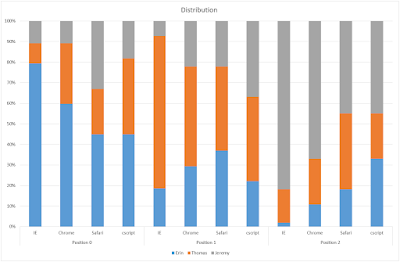distribution of results
But more interesting is who is first: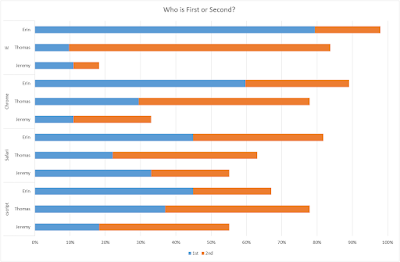How is first or second?
In this bar chart you can see (separated by browser) the percentages of who is first or at least second. For example on Internet Explorer, Erin is in 79% first, and in 98% at first or second. I would say that was a good cheating!

So yes, this code is causing highly biased results. Feel free to test it with more browsers.

It's also interesting that we can easily test which of the three browser categories yours is by using the sort function for fingerprinting:

This detection was done with a very simple javascript, testing just one specific case where all four act different: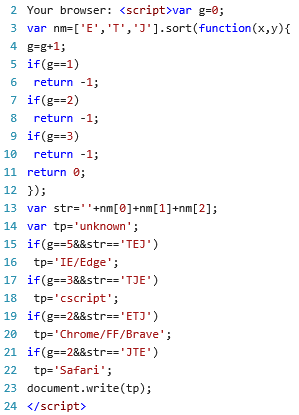simple fingerprinting source

Additionally I felt like writing some javascript that shows the entire table and creates a full fingerprint for all rows. The following table shows the sorting results for your current browser:

The fingerprint is the concatenated text string of the id for each row. The id is calculated like this: The five input values are treated like a base 3 number of five digits. This gives a maximum number of 243 (decimal). That number in hex are the first two digits of the id. Then we have the length of the input (usually 2..5, but theoretically it can also be 1 or 6) and one of six possible different outputs. These two values are combined into one more character.

Here's how the code looks like:actual calculation function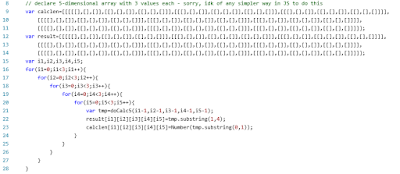loop over all 3^5 combinations and call calculationsimplify data (shorter sequences don't need all rows)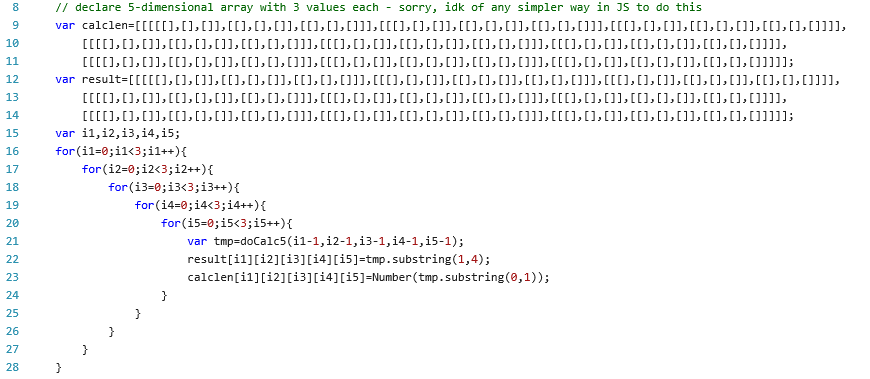output table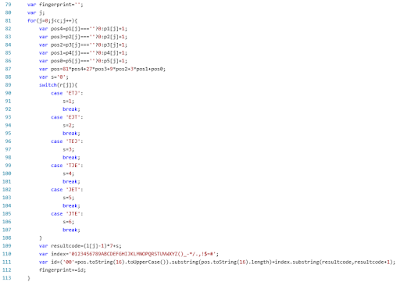calculate fingerprintprint the fingerprint data
If you need this, you can copy the text from view source, or ask me on Twitter if you want that I add it to GitHub.

Update from 2 October 2016: fixed some typos, added some charts, added javascript code for fingerprint string and dynamic table creation.